Next: Wavelength dependence Up: Extinction properties (transmitted radiation) Previous: Extinction properties (transmitted radiation)

## Extinction efficiencies: general behaviour and deviations

The extinction efficiency factorsare usually considered as a function of the size parameter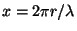for a fixed refractive indexthat, strictly speaking, does not characterize the dependencebecause of the wavelength dependence of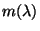(Sect. 2.1.2 in Voshchinnikov ). The behaviour of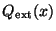is as follows: initially it rises rapidly with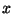, has several maxima and minima and goes asymptotically to a constant with a decaying oscillation. This behaviour is typical for weakly absorbing homogeneous spheres (Fig. 1, upper panel; see also Fig. 11 in Voshchinnikov ).The large-scale oscillations are caused by the interference between near forward transmission and diffraction (A.R. Jones, ). The height of the first peak is always maximal while subsequent peaks become lower and lower. The peak positions are determined by the values of the phase shift(the path of the central ray through the particle, see Sect. 2.2.3 in Voshchinnikov ). The efficiencies plotted against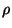have maxima at nearly the same positions for differentuntil the imaginary part of refractive index is small (see, e.g., Martin, ).

For large values of, the extinction factors approach the limiting value 2 (see Eq. (2.73) and Fig. 11 in Voshchinnikov ). This would suggest that the particle will block off twice the light falling upon it, an effect calling the extinction paradox'' (van de Hulst, ; Bohren and Huffman, ). Its explanation lies in the fact that two different phenomena are occurring: diffraction and the geometrical optics effects of reflection, refraction and absorption. The efficiency for each of these effects is one and henceIf the particle is very large, the diffraction pattern is very narrow. As laboratory detectors (including the human eye) collect light over a finite angular range, the diffracted light is registered and not measured as a loss. The measurement yieldsas would be expected. However, the conditions in astronomy totally differ from those in the laboratory and the diffracted light is always lost, i.e. the extinction paradox is present.

The ripple-like structure in the form of small extremely sharp peaks and troughs is observed for non-absorbing particles if the real part of the refractive indexis large (Fig. 1 as well as Fig. 11 in Voshchinnikov ). These ripple-like fluctuations result from the resonances of virtual modes (Bohren and Huffman, ). From the mathematical point of view, the scattering resonances are associated with zeros of the denominator in the expressions for Mie coefficients. The exclusion of the zeros lies in the basis of the S-approximation discussed in Sect. 2.2.4 in Voshchinnikov . Note that the ripples are washed away by any size distribution of particles.

Figure 1 illustrates the effect of variations of the real and imaginary parts of the refractive index and can be used for the prediction of extinction produced by real materials (e.g., given in Table 3 in Voshchinnikov ). In particular, it is seen that the largest extinction for a given spherical particle can be obtained for dielectric particles with large values of(silicates, quartz, MgO, SiC). At the same time, the extinction by metals or carbonaceous particles dominates for very small size parameters (Fig. 1 as well as Fig. 12 in Voshchinnikov ). The dilution of any material by the vacuum reduces bothand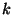(Table 4 and Fig. 5 in Voshchinnikov ), which causes a shift and decrease of interference maxima and smoothing of the extinction curves (Fig. 1).

The behaviour of extinction for spherical particles described above is considered as typical for extinction factors. The deviations from it occur when the inhomogeneous or/and non-spherical particles are examined. Inhomogeneity causes variations of refraction and reflection inside a particle that changes the optical path of the rays. For non-spherical particles, refraction and reflection on the surfaces with variable curvature result in alteration of scattering by such particles in the comparison with that by spheres.

The optical properties of core-mantle spheres were studied rather well and seems to show no significant peculiarities (Prishivalko et al., ) but already three-layered spheres can produce anomalous extinction of light. This is illustrated in Fig. 2 where the extinction efficiency factorsare plotted for spheres consisting of 3, 9 and 15 equivolume layers. The layers are composed of different materials (amorphous carbon (AC1), astrosil and vacuum) but the total volume fraction of each constituent is 1/3. The optical constants for AC1 and astrosil were taken as shown in Table 3 in Voshchinnikov . The peculiar behaviour of extinction is seen on the upper panel of Fig. 2 for the case of particles with the carbon core and astrosil as the outermost layer. Here, a very rare situation is observed when the first maximum is damped but there is very broad second maximum which is the highest. This peculiarity disappears if the number of layers increases: the difference in curves is hardly distinguished for particles with 15 layers (lower panel of Fig. 2). The given fact, noted by Voshchinnikov and Mathis (), allows one to suggest multi-layered particles as a new approximate model of composite grains. Such a model permits us to include an arbitrary fraction of any material and its numerical realization requires rather moderate computational resources (see Sect. 3.2.5 in Voshchinnikov ).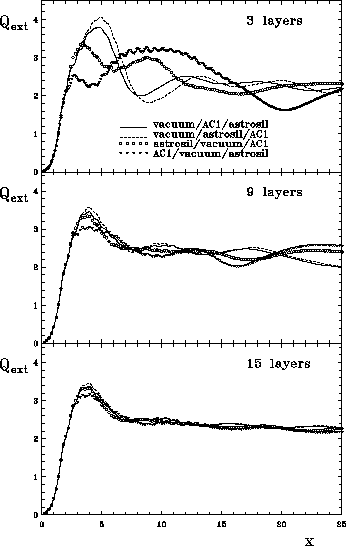The dependence of the extinction efficiency factoron the parameter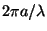for prolate and oblate spheroids with the refractive indexand the aspect ratio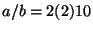is shown in Figs. 3 and 4. The normalization used in Fig. 4 gives the possibility of comparing the factors calculated for different orientations of a particle. Large-scale variations of the factor have the same reasons as for spheres. But some features of the behaviour of the factors plotted in Figs. 3a and 4b take place: the third maximum is higher than the second one for prolate spheroids at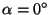and for oblate spheroids atif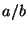= 4 and 6. Asano () found the similar peculiarity for particles withand 5. This phenomenon is determined by the particle shape and orientation and appears for dielectric particles with different ratios(see also Voshchinnikov, ).As seen from Fig. 3a, for prolate spheroids the values ofcan be rather large if radiation propagates along the major (rotation) axis of a particle (). This is a result of normalization by the geometrical cross-section which is small in this case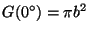(see Eq. (2.41) in Voshchinnikov ). The factors can be even larger for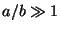(see Fig. 5).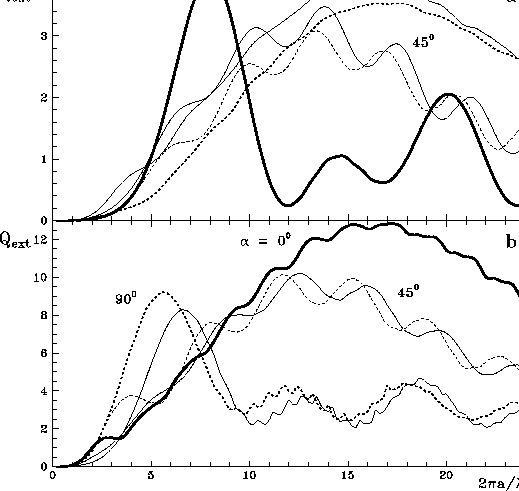As follows from this Figure, the behaviour of the factors for very elongated spheroids is rather regular, and the values ofsmoothly decrease with the size parameter. There are 25 maxima on the interval0-300, which is totally distinct from the behaviour for spherical particles (cf. Fig. 11 in Voshchinnikov ). Note that the size of equivolume'' particles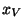considered in Fig. 5 is moderate: from Eq. (2.39) in Voshchinnikov  it follows that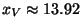if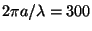and. But the path of light inside a spheroidal particle is in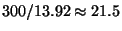times longer than inside a sphere of the same volume.

It is significant that the limiting value of the extinction factors for particles of any shape must be equal to 2 but as is seen from the inset in Fig. 5 this condition is far for being satisfied although the tendency to reduction ofis observed.

Figures 3 and 4 also show that ripple-like fluctuations are better seen for the oblate spheroids than for the prolate ones. The ripples vanish from sight for elongated (prolate) spheroids if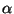approaches zero. A similar picture is observed for infinite cylinders (Fig. 6). In this Figure, the extinction efficiencies for prolate spheroids and infinitely long circular cylinders are compared.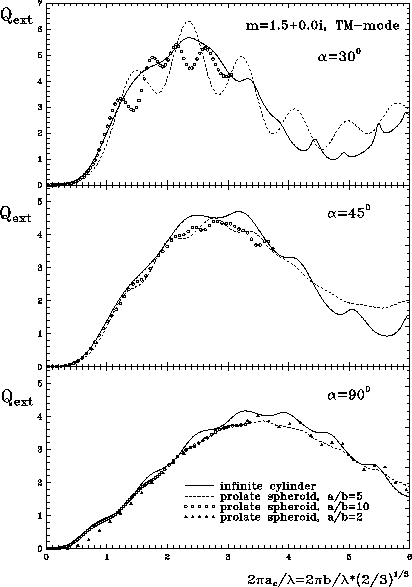The simplest model of non-spherical particles -- infinite circular cylinders -- is not physically reasonable. However, it looks attractive to find cases when this model could be useful because calculations in this case are very simple and fast. Previous attempts to find the limits of applicability of the model of infinite cylinders were made by Martin () and Voshchinnikov (). In both cases, particles of the same thickness were considered, i.e. spheroids and cylinders had the equal size parameters. Martin () notes that the factors for spheroids resemble those for cylinders iffor the normal incidence of radiation () but in order to align the peaks in extinction, the-scale for cylinders was stretched by a factor 1.13.

Voshchinnikov and Farafonov () established a similarity of the behaviour of the efficiency factors: both TM and TE-modes for spheroids converge to some limiting values which are close to those of infinite cylinders. This occurs if the size parameter is defined in a special way -- both the volume and the aspect ratio of a spheroid and a very long cylinder with the length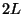are put to be the same, i.e.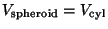and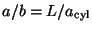. So, we need to compare the particles with size parametersandas it is shown in Fig. 6. Note that the scaling factor [] which arises is close to that empirically found by Martin (). For the normal and oblique incidence of radiation (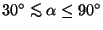), the efficiency factors converge to some limit values with an increase of the aspect ratio, provided spheroids of the same volume and thickness are considered. These values are close to, but do not coincide with the factors for infinite cylinders (see Voshchinnikov and Farafonov,  for more details).Next: Wavelength dependence Up: Extinction properties (transmitted radiation) Previous: Extinction properties (transmitted radiation)
root 2003-04-09